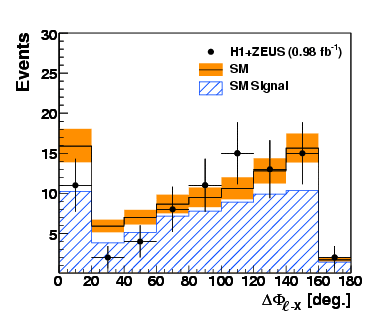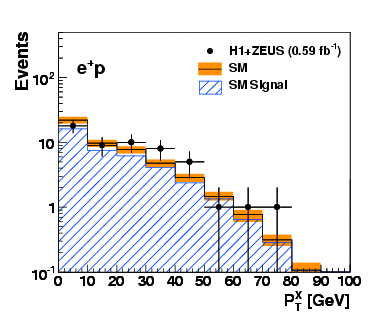H1Zeus - HERA Combined Results# Contacts: James Ferrando (ZEUS), David South (H1) Production of isolated leptons associated with missing transverse momentum has a very low cross section in the SM, dominated by real W boson production with subsequent leptonic decay.

### H1 + ZEUS combined results:

Events with an Isolated Lepton and Missing Transverse Momentum and Measurement of W Production at HERA Published in JHEP 1003 (2010) 035 (1) Distribution of lepton polar angleDistribution of lepton polar angle

(2) Distribution of the difference in the azimuthal angle of the lepton and the hadronic systemDistribution of the difference in the azimuthal angle of the lepton and the hadronic system

(3) Transverse MassTransverse Mass

(4) Hadronic transverse momentum(5) Missing transverse momentumMissing transverse momentum

(6) Lepton transverse momentumLepton transverse momentum

(7) Hadronic transverse momentum for e+p dataHadronic transverse momentum for e+p data

(8) Hadronic transverse momentum for e-p dataHadronic transverse momentum for e-p data

(9) Single W production cross section as a function of PT of the hadronic systemSingle W production cross section as a function of PT of the hadronic system